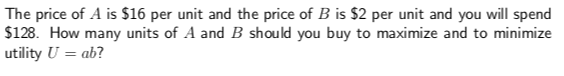The price of A is \$16 per unit and the price of B is \$2 per unit and you will spend\$128. How many units of A and B should you buy to maximize and to minimizeutility Uab?

Questionhelp_outlineImage TranscriptioncloseThe price of A is \$16 per unit and the price of B is \$2 per unit and you will spend \$128. How many units of A and B should you buy to maximize and to minimize utility Uab? fullscreen
Step 1

It is given that the price of A is \$16 per unit and the price of B is \$2 per unit. The amount to spend is \$128. Assume that the number of units of A and B are x and y, respectively.

Then, the equation can be formulated as

Step 2

Here, the utility function is given as

Step 3

Obtain the value of y and substitute y in...

Want to see the full answer?

See Solution

Want to see this answer and more?

Our solutions are written by experts, many with advanced degrees, and available 24/7

See Solution
Tagged in

Derivative